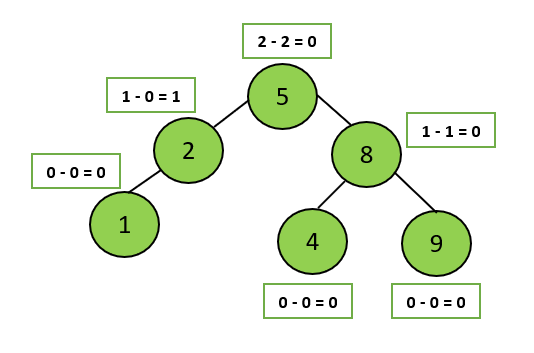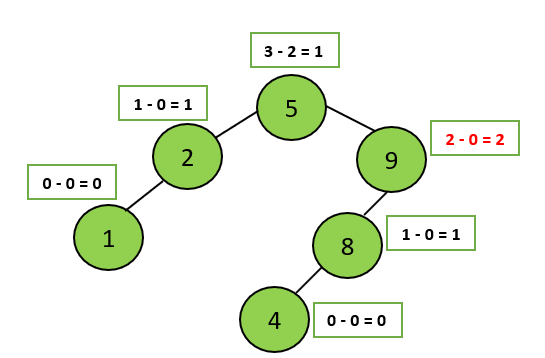# Deletion in AVL Tree

## Introduction

Adelson-Velskii and Landis (AVL) is a type of Binary Search Tree. In the AVL tree, the difference between the height of the left and right subtree is at most 1. This difference is known as the balance factor.

1. Introduction to AVL Trees
2. Insertion in AVL Tree
3. Deletion in AVL Tree

## AVL Tree Properties

A binary tree is an AVL tree, if:

1. It is a binary search tree, and
2. For any node A, the height of the left subtree of A and the height of the right subtree of A differ by at most 1.Figure 1 Figure 2

For example, among the above binary search trees, Figure 1 is an AVL tree, whereas Figure 2 is not. The reason is, in figure 2, the node having value 9 has a balance factor of 2.

## Modification/ Deletion in an AVL Tree

When we insert or delete an element in a tree, its structure changes. To restore the AVL tree property, we need to modify the tree. This can be done using rotations.

An insertion or deletion involves adding or deleting a single node. This can only increase or decrease the height of a subtree by 1. After every operation, the AVL tree can be restored by using single or double rotation. To revise the concept of rotation in AVL trees do visit here.

## Algorithm

AVL Tree is a self-balancing binary search tree. Deletion of a node will be the same as in BST. After a node has been deleted according to BST, we’ll check for the unbalanced nodes(Nodes having a balance factor of more than 1).

The remaining steps are as follows:

1. After the node is deleted, start from its parent, move up to the tree's root, and check for the first unbalanced node.
2. Restore the AVL tree property by performing one of the following rotations.

To know more about rotations and how they are performed, you can visit this amazing article on Introduction to AVL Trees.

Let’s understand what is the basic difference between the two main operations i.e. Insertion and Deletion.

## Difference between Insertion and Deletion in AVL Tree

Insertion: After an insertion, subtrees of the node are changed, resulting in the change of balance factor in the path from the point of insertion to the root. We need to restore the AVL tree property.

We need to consider the first node with a balance factor greater than one while moving to the root. Starting from this node, every node till the root will have the issue.

If we fix the issue here, then all other nodes will automatically satisfy the AVL tree property.

For learning more about insertion in AVL trees and their Implementation, refer to Part-2 of the series.

Deletion:

In contrast to insertion, after performing a rotation at a node to restore the AVL property, we may need to perform a rotation at that node's ancestors. As a result, we must follow the path until we reach the root.

Let’s understand this with the help of an example.

## Understand deleting a node from the AVL tree with the help of an example

Given an AVL Tree:

Step 1: Deleting node 0025.

The tree will become this:

This is not balanced(the node 0040 has a balanced factor of 2), Hence, Not an AVL tree.

Step 2: Start from 0030, move till the root, i.e. 0040. Find the first unbalanced node.

The first unbalanced node is 0040(Having a balance factor of 2).

Step 3: Perform rotation to make it balanced.

This is a balanced AVL Tree.

Output:

## Complexity Analysis

Time Complexity:

The time complexity of an AVL delete is the same as that of a BST delete, which is O(h), where h is the height of the tree. Rotation, updating the height and getting the balance factor takes constant time.

The height of the AVL tree is O(logn) since it is balanced. As a result, the AVL delete has an O(log n) time complexity.

Space Complexity:

For deleting an element, we have to traverse the tree to find it. Space complexity depends on the number of stack frames required in the recursive traversal.

The space complexity of a recursive in-order traversal is O(h), where h is the height of the tree. The height of the AVL tree is O(logn) since it is balanced. As a result, the AVL delete has an O(log n) space complexity.

1. What is the maximum height of an AVL tree having n nodes?
Answer: The maximum height of an AVL tree having n nodes is log(n).

2. State two properties of AVL trees.Answer:
Answer: The important properties of an AVL tree are:
→ It is a binary search tree,
→ andFor any node A, the height of the left subtree of A and the height of the right subtree of A differ by at most 1.

3. What is the balancing condition of an AVL tree?
Answer: The balancing condition of an AVL tree is:
balance(n) = abs(height(n.left)−height(n.right))

4. What is the time complexity of deleting a node from the AVL tree?
Answer: Due to the balancing property, the deletion operations take O ( l o g n ) time in both the average and the worst cases.

## Key Takeaways

Here's a treat for you, you can create your AVL tree by inserting and deleting nodes virtually. You can also see how these rotations work on this fantastic AVL Tree Virtualization webpage.

In this article, we learned about deleting a node from the AVL tree along with its proper implementation in C++.

To get a complete insight into AVL trees, we recommend reading this series on AVL trees.

Happy Learning!